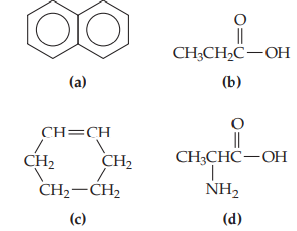×
Get Full Access to Chemistry: The Central Science - 12 Edition - Chapter 24 - Problem 3e
Get Full Access to Chemistry: The Central Science - 12 Edition - Chapter 24 - Problem 3e

×

# Which of these molecules most readily undergoes anISBN: 9780321696724 27

## Solution for problem 3E Chapter 24

Chemistry: The Central Science | 12th Edition

• Textbook Solutions
• 2901 Step-by-step solutions solved by professors and subject experts
• Get 24/7 help from StudySoup virtual teaching assistantsChemistry: The Central Science | 12th Edition

4 5 1 426 Reviews
25
3
Problem 3E

Which of these molecules most readily undergoes an addition reaction? [Section 24.3]Step-by-Step Solution:
Step 1 of 3

14.4 THE CHANGE OF CONCENTRATION WITH TIME First-Order Reactions - first-order reaction – one whose rate depends on the concentration of a single reactant raised to the first power - if the rxn of the type A products is first order, the rate law is Rate = - ∆ A = k [A] ∆t - this form, which expresses how rate depends on concentration, is called differential rate law - this relationship can be transformed into an eqn known as integrated rate law for a first-order rxn that relates the initial concentration of A, [A] 0 to its concentration at any other time t, [A] t ln[A] t ln[A] =0-k t or [A] ln t = -k t [A] 0 or ln[A] t -k t + ln[A] 0 y = mx + b ^ for first-order rxns, which will result in a straight line Second-Order Reactions - second-order reaction – rate depends on either a reactant concentration raised to the second power or on the concentration of two reactants each raised to the first power - consider A products or A+ B  products ∆A 2 Rate = - ∆t = k [A]

Step 2 of 3

Step 3 of 3

##### ISBN: 9780321696724

Unlock Textbook Solution